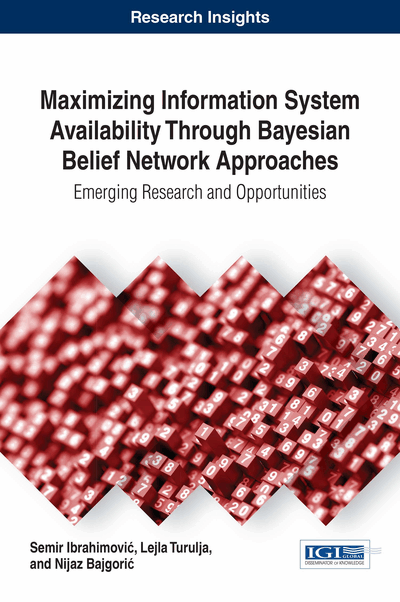# Bayesian Belief Networks in IT Investment Decision Making

DOI: 10.4018/978-1-5225-2268-3.ch004
Available
\$29.50
No Current Special Offers

## Abstract

This section aims at describing the concept of Bayesian Belief Networks (BBN), building principles and application of BBN and influence diagrams, as well as the reasons why BBN are considered an adequate tool for IS availability modeling.
Chapter Preview
Top

## Bayesian Belief Networks (Bbn)

The main reason for choosing this instrument is its applicability in the treatment of problems of this domain. While writing about the application of BBN on modeling operational risk information technology in financial institutions Neil, Marquez, & Fenton (2008) argued that the main advantages of BBN are in enabling a combination of statistical and qualitative data and mapping the causal structure of the process, thus making it easier to understand and communicate with business users. BBN method enables combining proactive loss indicators with reactive consequence measurement, considering experts’ judgments using a qualitative risk assessment, working with incomplete data and still get a reasonable prediction. Besides that, by using BBN one can implement strong scenario analysis, test the robustness of the results, have a tool for visual reasoning and help in documentation, conduct a comparative analysis of alternative scenarios and check the robustness of the IT infrastructure on the changes in the design.

BBN are graphical models that combine the graph and probability theory. Each BBN consists of two elements: directed acyclic graphs representing the structure, and a set of conditional probability tables -CPT. The nodes in the structure correspond to the variables of interest, and the edges are interpreted in terms of conditional probabilities according to Bayes theorem. For many practical applications, an edge represents a direct causal effect.

BBN must contain CPT for each variable which quantifies a relationship between the variable and its “parent” in the graph (Darwiche, 2010). Bayesian network can be used to answer probabilistic questions about the variables and their relationships represented by the network. For example, a network can be used to find out, by using Bayes theorem, an updated knowledge of the state of a variables subset when other variables (the evidence variables) are observed. This process of computing the posterior distribution of variables given evidence is called probabilistic inference. A Bayesian network can thus be considered a mechanism for automatically applying Bayes’ theorem to complex problems (Ding, 2010). For two events, Bayes' theorem is represented as follows:

(19)

This formula means that it is possible to calculate the conditional probability of event A, for a given event B, using conditional probabilities of event B, for a given event A and unconditional probabilities of event A and event B. In the case that states of any nodes are fixed, Bayes' theorem can be used for propagation in backward direction, and the thus calculate posterior probabilities for every node in the graph. This is the basic scenario in BBN analysis (Carol, 2003). BBN can be used in three ways:

• Causation Reasoning: Calculation of probabilities that consequence is caused by the occurrence of the particular event (probability that cancer is caused by smoking).

• Diagnostic Reasoning: Search probability that the consequence will happen if a particular event occurs, (probability that someone will get cancer if he smokes).

• Support Reasoning: Search the interaction among the causes (Yu, Zheng, & Qian, 2009).

## Complete Chapter List

Search this Book:
Reset